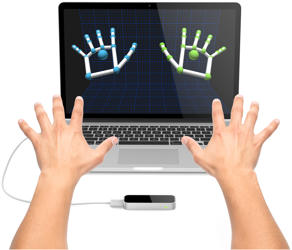# Gesture Interactions# Using leap motion

https://www.leapmotion.com/setup
2. Open the processing library manager from the context menu:
Sketch > Import Library > add Library
3. Search for and install “Leap Motion for Processing” in the “Library Manager” dialogue box.
4. https://github.com/voidplus/leap-motion-processing

Gesture Interaction References:

https://developer.leapmotion.com/documentation/java/devguide/Leap_Overview.html

Minority report

# Basic hand Location

```import de.voidplus.leapmotion.*;

LeapMotion leap;

void setup(){
size(1000, 800);
leap = new LeapMotion(this);
}

void draw(){
background(255);

// ========= get hand locations from Leapmotion =========
for(Hand hand : leap.getHands()){

// ----- Variables -----
PVector hand_position    = hand.getPosition();
boolean hand_is_left     = hand.isLeft();
boolean hand_is_right    = hand.isRight();

if(hand_is_left) {
// println("left"+hand_position);
fill(0,0,255);
ellipse(hand_position.x, hand_position.y, 16, 16);
}
if(hand_is_right) {
fill(255,0,0);
//println("right"+hand_position);
ellipse(hand_position.x, hand_position.y, 16, 16);
}
}
}```

# Finger and Joint Locations

```import de.voidplus.leapmotion.*;

LeapMotion leap;

void setup() {
size(800, 500);
leap = new LeapMotion(this);
}

void draw() {
background(255);

// ========= HANDS =========

for (Hand hand : leap.getHands ()) {
// ----- SPECIFIC FINGER POINT -----

Finger  finger_thumb     = hand.getThumb();
PVector  finger_thumb_loc  = finger_thumb.getPosition();

Finger  finger_index     = hand.getIndexFinger();
PVector finger_index_loc  = finger_index.getPosition();

Finger  finger_middle    = hand.getMiddleFinger();
PVector finger_middle_loc  = finger_middle.getPosition();

Finger  finger_ring      = hand.getRingFinger();
PVector finger_ring_loc = finger_ring.getPosition();

Finger  finger_pink      = hand.getPinkyFinger();
PVector finger_pink_loc  = finger_pink.getPosition();

// ----- DRAWING -----

drawEllipse(finger_thumb_loc);
drawEllipse(finger_index_loc);
drawEllipse(finger_middle_loc);
drawEllipse(finger_ring_loc);
drawEllipse(finger_pink_loc);

// ========= Joints =========

for (Finger finger : hand.getFingers()) {

// ----- SPECIFIC BONE -----

Bone bone_distal = finger.getDistalBone();

Bone bone_intermediate = finger.getIntermediateBone();

Bone bone_proximal = finger.getProximalBone();

Bone bone_metacarpal = finger.getMetacarpalBone();

// ----- DRAWING -----

finger.drawJoints();
finger.drawLines();

}
}
}

void drawEllipse(PVector point) {
ellipse(point.x, point.y, 10, 10);
}```

# Swipe Recognition

```import de.voidplus.leapmotion.*;

LeapMotion leap;

void setup(){
size(800, 500);
background(255);
// leap = new LeapMotion(this).withGestures(); // with all gestures
leap = new LeapMotion(this).withGestures("swipe"); // Leap detects only swipe gestures.
}

void draw(){
}

// ----- SWIPE GESTURE -----

void leapOnSwipeGesture(SwipeGesture g, int state){

int  id                  = g.getId();
PVector position_start      = g.getStartPosition();
PVector position            = g.getPosition();

switch(state){
case 1: // Start
break;
case 2: // Update
break;
case 3: // Stop
position.sub(position_start);
position.normalize();
if(position.x >= 0) {
println("towards right");
} else {
println("towards left");
}
break;
}
}```